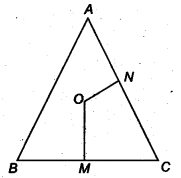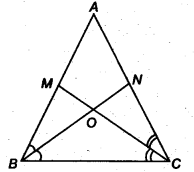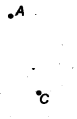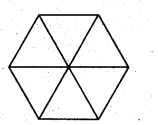NCERT Solutions for Class 9 Maths Chapter 5 Triangles Ex 5.5 are part of NCERT Solutions for Class 9 Maths. Here we have given NCERT Solutions for Class 9 Maths Chapter 5 Triangles Ex 5.5.

 Board CBSE Textbook NCERT Class Class 9 Subject Maths Chapter Chapter 5 Chapter Name Triangles Exercise Ex 5.5 Number of Questions Solved 4 Category NCERT Solutions

## NCERT Solutions for Class 9 Maths Chapter 5 Triangles Ex 5.5

Question 1.
ABC is a triangle. Locate a point in the interior of ∆ ABC which is equidistant from all the vertices of ∆ ABC.
Solution:
Suppose OM and ON be the perpendicular bisectors of sides BC and AC of ∆ ABC.So, O is equidistant from two endpoints 0 and C of line segment BC as O lies on the perpendicular bisector of BC. Similarly, O is equidistant from C and A Hence, O be an orthocentre of ∆ABC.

Question 2.
In a triangle locate a point in its interior which is equidistant from all the sides of the triangle.
Solution:
Suppose BN and CM be the bisectors of ∠ ABC and ∠ ACB, respectively intersect AC and AB at N and M, respectively.Since, O lies on the bisector BN of ∠ ABC, so O will be equidistant from BA and BC. Again, O lies on the bisector CM of ∠ ACB.
So, O will be equidistant from CA and BC. Thus, O will be equidistant from AB, BC and CA Hence, O be a circumcentre of ∆ABC.

Question 3.
In a huge park, people are concentrated at three points (see figure)
A: where these are different slides and swings for children.
B: near which a man-made lake is situated.
C: which is near to a large parking and exist.
Where should an ice-cream parlor be set? up so that maximum number of persons can approach it?
[Hint The parlour should be equidistant from A, B and C.]Solution:
The ice-cream parlor should be equidistant from A B and C for which the point of intersection of perpendicular bisectors of AB, BC, and CA should be situated.
So, O is the required point which is equidistant from A B and C.

Question 4.
Complete the hexagonal and star shaped Rangolies [see Fig. (i) and (ii)] by filling them with as many equilateral triangles of side 1 cm as you can. Count the number of triangles in each case. Which has more triangles?Solution:
We first divide the hexagon into six equilateral triangles of side 5cm as follow.We take one triangle from six equilateral triangles as shown above and make as many equilateral triangles of one side 1 cm as shown in the figure.The number of equilateral triangles of side 1 cm = 1 + 3 + 5 + 7 + 9 = 25
So, the total number of triangles in the hexagon = 6x 25 = 150
To find the number of triangles in the Fig. (ii), we adopt the same procedure.
So, the number of triangles in the Fig. (ii) = 12 x 25 = 30Q Hence, Fig. (ii) has more triangles.
We hope the NCERT Solutions for Class 9 Maths Chapter 5 Triangles Ex 5.5 help you. If you have any query regarding NCERT Solutions for Class 9 Maths Chapter 5 Triangles Ex 5.5, drop a comment below and we will get back to you at the earliest.